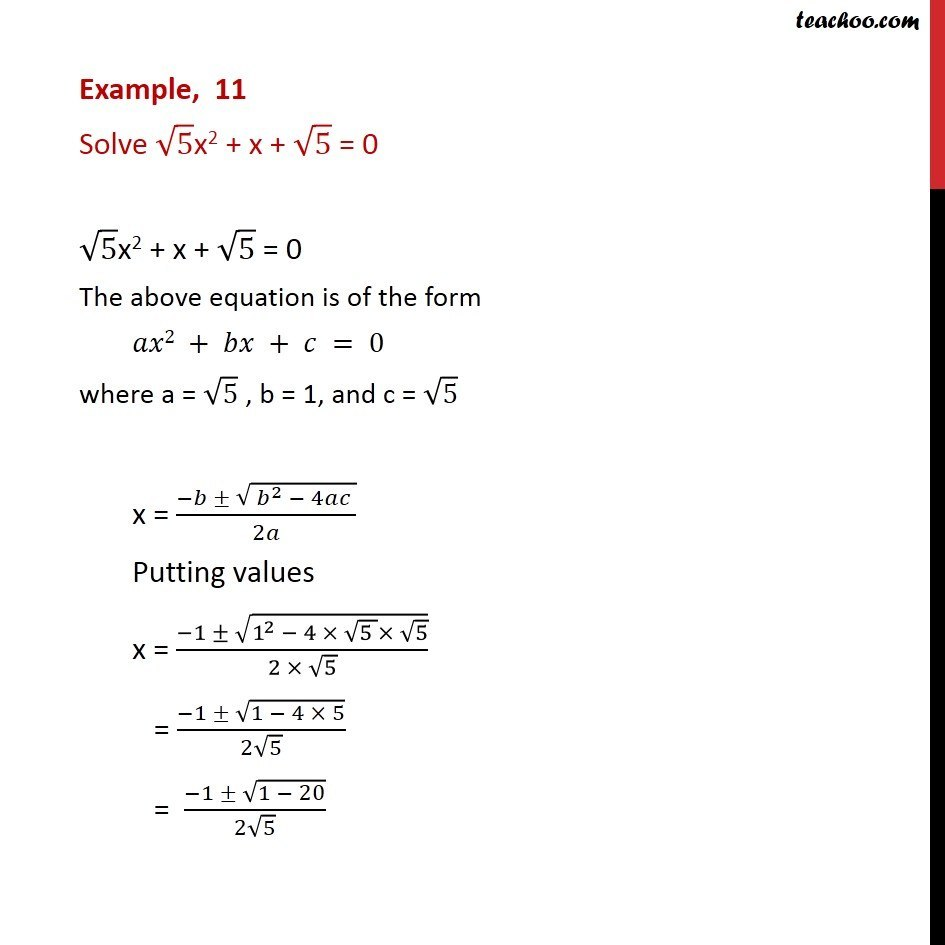Examples

Chapter 4 Class 11 Complex Numbers
Serial order wiseLearn in your speed, with individual attention - Teachoo Maths 1-on-1 Class

### Transcript

Example, 11 Solve 5x2 + x + 5 = 0 5x2 + x + 5 = 0 The above equation is of the form 2 + + = 0 where a = 5 , b = 1, and c = 5 x = ( ( ^2 4 ))/2 Putting values x = ( 1 (1^2 4 (5 ) 5) )/(2 5) = ( 1 (1 4 5))/(2 5) = ( 1 (1 20))/(2 5) = ( 1 ( 19))/(2 5) = ( 1 19 ( 1))/(2 5) = ( 1 19 )/(2 5) Thus, = ( 1 19 )/(2 5)